# COSIN/io 2D Road Type: sine_sweep

A sine-shaped road profile with slowly varying frequency and amplitude is calculated in two different ways:

## Linear sweep

The frequency increases linearly with respect to travel distance. The road height value z(s) as function of travel distance s is calculated as follows: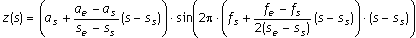Note: The factor 2 in the denominator is not an error!

The actual frequency (= derivative of the sine function argument with respect to travel path, divided by 2π; this is not equal to the factor that is multiplied by in the sine function!) is given by: 2π(s - ss)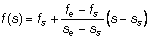## Logarithmic sweep

The wave length decreases by a constant factor with every cycle. The road height value is calculated as follows: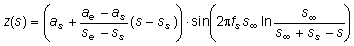where,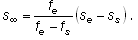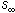is the travel path that theoretically an infinitely high frequency is reached, measured relative to sweep start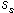. The actual frequency is given by: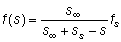For data supply, COSIN/road searches for the data block \$sine_sweep, and reads:
Name of input variable Unit Meaning
start m Start of swept sine wave (travel distance).
end m End of swept sine wave (travel distance)
amplitude_at_start mm Amplitude of swept sine wave at start.
amplitude_at_end mm Amplitude of swept sine wave at end.
wave_length_at_start m Wave length of swept sine wave at start.
wave_length_at_end m Wave length of swept sine wave at end. Must be less than or equal to wave_length_at_start.
sweep_type 0/1
0
Frequency changes linearly with respect to travel distance.
1
Wave length changes each cycle by a constant factor.